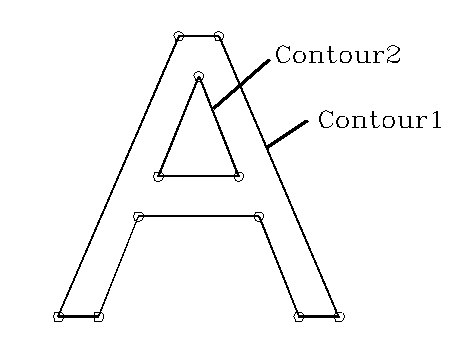Draw Contour Set

Purpose

The Draw Contour Set function draws a set of complex polygonal shaded regions.

Syntax

 Opcode format Opcode [ASCII] (Hex) Operand Format Comments Extended ASCII (ContourSet []+,[,]+ Absolute coordinates. Single-byte, binary operand [k] (71) [][[USP-Ecounti>]]+[]+ Relative coordinates. [Ctrl-k] (11) [][[USP-Ecounti>]]+[]+ Relative coordinates.

CS-count This is the number of contours in the contour set and it must be greater than zero. However, in the binary operand form, a value of zero for CS-count indicates that an extended count (CS-Ecount) will follow (as an unsigned short).

CS-Ecount When CS-count is zero, a 2-byte extended count follows allowing for values from 256 through 65,791 which are encoded as an integer (0 to 65,535).

P-counti The number of points in the ith contour. Must be 2 or greater. In the binary operand form, a value of zero for P-count indicates that an extended count (P-Ecount) will follow

P-Ecount When P-count is zero, a 2-byte extended count follows allowing for values from 256 through 65,791 which are encoded as an integer (0 to 65,535).

X1,Y1 The first point (in logical coordinates) in the first contour.

Xj,Yj The jth point (in logical coordinates) in the nth contour.

Notes

Figure 1 shows a contour set example, representing the letter "A", that could be used for transmitting text in an outline font format. In the figure, CS-count=2, P-count1=8 and P-count2=3.Figure 1. Contour set

Implementation in WHIP! Versions

 Toolkit Function TBD Partial Full Revised in Draw Contour Set - - 2.0 -